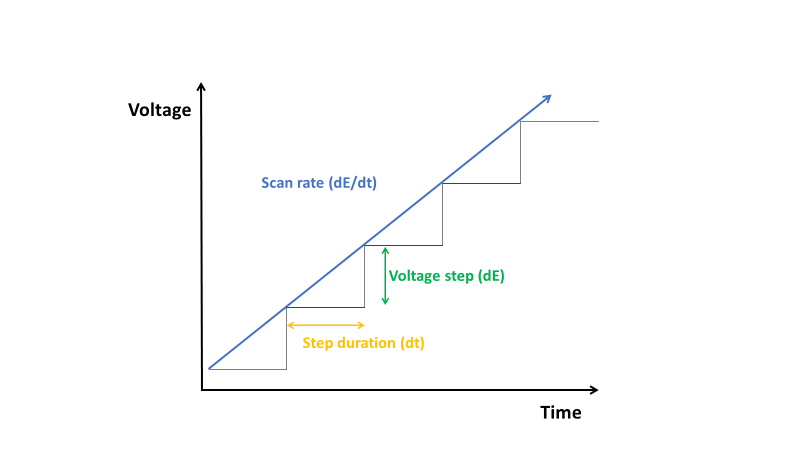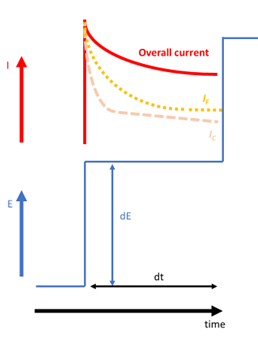Topic 5 min readCyclic Voltammetry: how to obtain great results with your potentiostat (Electrochemistry basics series)

Latest updated: November 19, 2021

Cyclic Voltammetry (CV) is the most widely used technique for acquiring qualitative information for electrochemical reactions. It is a voltamperometric technique available in the technique library of BioLogic potentiostat / galvanostats as well as BioLogic battery cyclers. The Cyclic voltammetry technique is used for battery testing as well as for electrochemical research, most notably for corrosion applications. Cyclic Voltammetry provides information on redox processes, heterogeneous electron-transfer reactions, and adsorption processes. It enables the user to rapidly identify the redox potential of the electroactive species and kinetic information. In simple terms, in Cyclic Voltammetry, the cell is stressed by a voltage scan, and the resulting current is then measured.

Although it is used on a daily basis, some parameters used in Cyclic Voltammetry have an effect on measurement quality and need to be clarified.

At what voltage should you start?

We recommend you start the measurement at the Open Circuit Voltage (OCV). This will keep the cell in its initial state. The cell will not be disturbed before the voltage scan. In the EC-Lab® Cyclic Voltammetry parameters setting window, the initial voltage is named $E_{\mathrm{i}}$ and is settle at 0.000 V vs. $E_{\mathrm{OC}}$ (open circuit potential before scan) by default.

How is the voltage scan performed by the potentiostat?

Nowadays, potentiostat / galvanostats are built using digital technology, so the voltage scan is carried out in successive voltage steps ($\mathrm{d}E$). In order to mimic a linear scan, the voltage step, dE, has to be as small as possible. The limiting factor is the voltage control resolution of the potentiostat or of the battery cycler.The controlled voltage resolution for a potentiostat can be improved by using a narrow voltage range. For example, the voltage resolution of a BioLogic VMP3 multichannel potentiostat is about 300 µV with a voltage range of +/-10 V and a 16 bit DAC. It can be improved by a factor of 3 by setting a voltage range of +/-2.5 V to obtain a resolution of around 100 µV.

Moreover, in order to smooth out the current signal, we recommend you average the current response over several steps. Because of the accuracy of the reference electrode which is around +/-1 mV, one data point every 1 mV is a good target.

That’s why, for electroanalytical applications in solution, we recommend you set your potentiostat with a voltage range of +/-2.5 V and average the current response over 10 steps.

NOTE: Some of our potentiostat / galvanostats offer the possibility to apply a “true” analog ramp (see premium’s ARG Analog Range Generator option).

How the current is sampled in Cyclic Voltammetry

The current is sampled along the whole voltage step. The potentiostat samples an overall current that is the sum of the capacitive, $I_{\mathrm{C}}$, and faradaic current, $I_{\mathrm{F}}$. As the decay of the capacitive current is faster than the faradaic current after the pulse (see equations (1) and (2)), depending on where the current is sampled, the contribution of the faradaic current will be different on the measured overall current of the electrochemical system.

The faradaic current is given by the following equation:

$I_\mathrm{F}=nFAC\sqrt{\frac{D}{\pi{t}}}\tag{1}$

Where $n$ is the number of electrons involved in the redox process, $F$ the Faraday constant, $A$ the surface of the electrode, $C$ the concentration of the electroactive species, $D$ the diffusion coefficient of the electroactive species, $t$ the time after the application of the pulse at which the current is sampled.

The capacitive current is given by the following equation:

$I_\mathrm{C}=\frac{E}{R}\mathrm{exp}\biggl(\frac{-t}{RC_{\mathrm{dl}}}\biggr)\tag{2}$

With $E$ the pulse potential, $R$ the ohmic resistance between the working electrode and the reference electrode, $C{\mathrm{dl}}$ the double-layer capacitance.Typically, 50% of the end of the step is a good setting for electroanalytical purposes. If the user is also interested in obtaining a larger capacitive current, a wider step can be set.

TIP: it is possible to remove the capacitive current by subtracting the Cyclic Voltammetry of the blank (performed in the same condition i.e. same electrode, same voltage sweep.) from the Cyclic voltammetry of interest.

Current ranging…

During the shift, from one current range to another, you will see a transient current. Because of the transient nature of this current, it does not make sense to record these data. It will induce a peak that will make post-processing more difficult to manage. This is the reason why BioLogic potentiostats do not record these points.

For scan rates faster than hundreds of mV/s, we recommend you set a fixed current range so you will not miss any points.

Ohmic drop compensation

The cyclic voltammetry technique can be associated with a technique that compensates for the Ohmic Drop. Impedance measurements at high frequencies allow the determination of the ohmic drop of an electrochemical system. The ZIR EC-Lab® and BT-Lab® techniques are Ohmic Drop Determination techniques using PEIS (Potentio Electrochemical Impedance Spectroscopy). Coupled with the cyclic voltammetry technique these techniques enable users to perform electrochemical experiments, freeing themselves from limitations caused by ohmic drop influence. See application notes 27-29.

Bandwidth

More information can be found in « Understanding bandwidth & its effect on measurements » but in simple terms, the faster you scan, the faster the bandwidth should be.

Application note 27: Ohmic drop effect on measurements

Application note 28: Ohmic drop measurement techniques

Application note 29: Ohmic drop & ZIR techniques

CV Cyclic Voltammetry Voltage scan

Related products

Work smarter. Not harder.

Tech-tips, theory, latest functionality, new products & more.

No thanks!